# Reverse tree path

Given a tree and a node data, your task to reverse the path till that particular Node.

Examples:

```Input :
7
/    \
6       5
/ \     / \
4  3     2  1
Data = 4
Output : Inorder of tree
7 6 3 4 2 5 1

Input :
7
/    \
6       5
/ \     / \
4  3     2  1
Data = 2
Output : Inorder of tree
4 6 3 2 7 5 1
```

## Recommended: Please try your approach on {IDE} first, before moving on to the solution.

The idea is to use a map to store path level wise.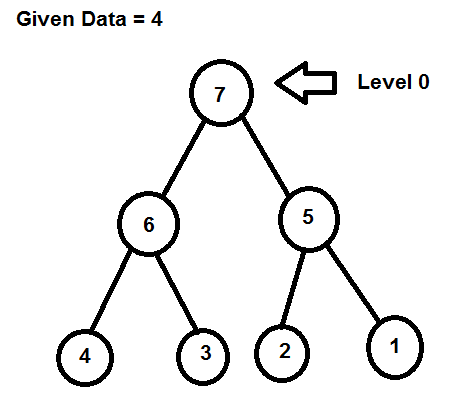Find the Node path as well as store in the map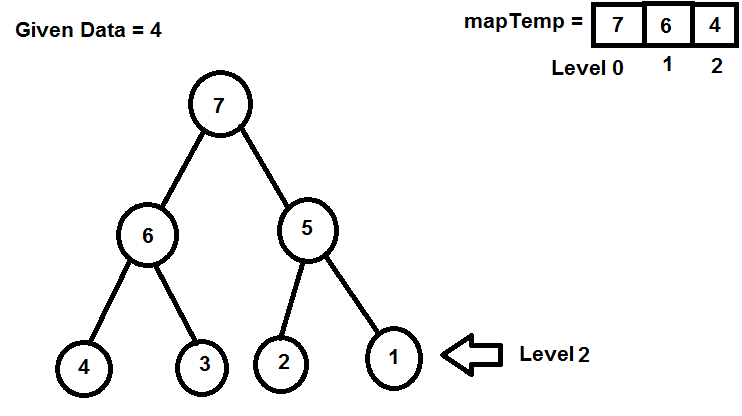the path is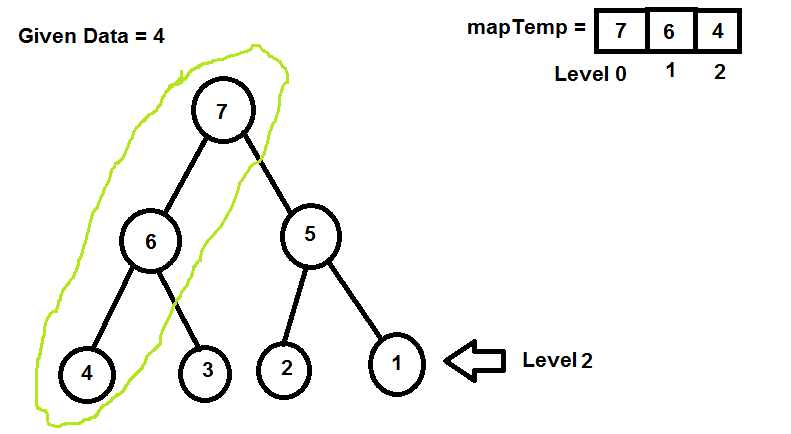Replace the position with the map nextPos index value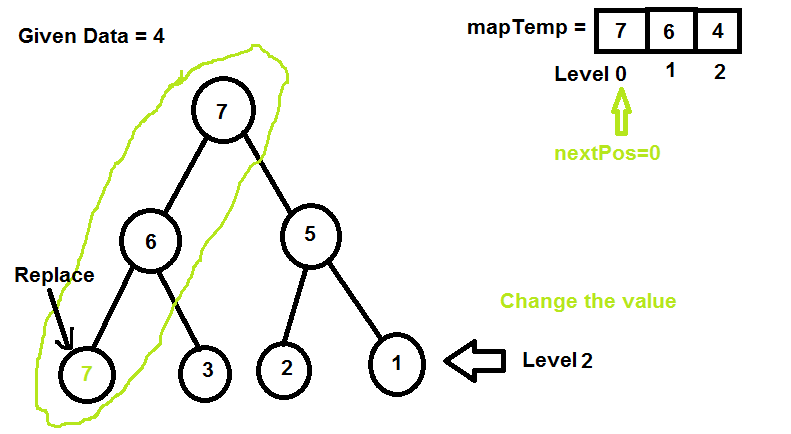increment the nextpos index and replace the next value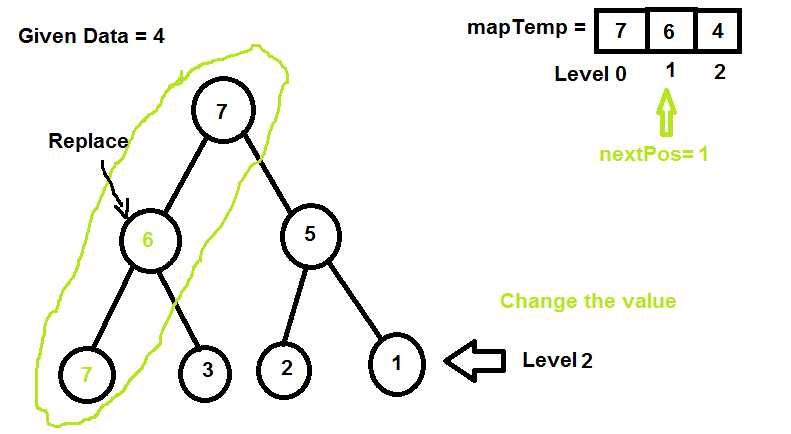increment the nextpos index and replace the next value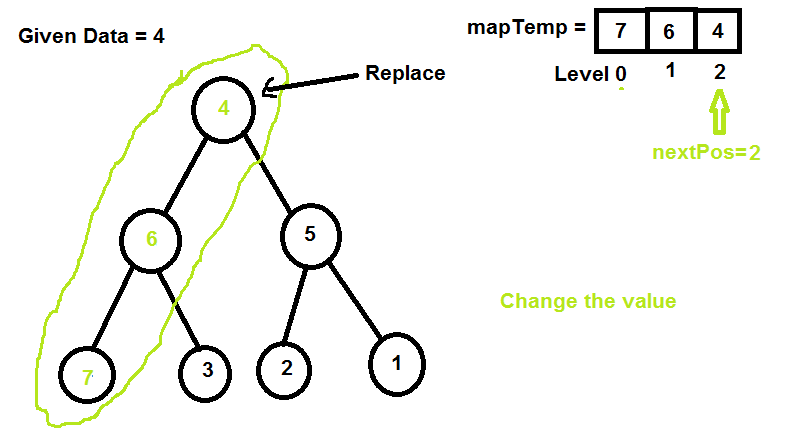Let’s understand with the code.

## C++

 `// CPP program to Reverse Tree path ` `#include ` `using` `namespace` `std; ` ` `  `// A Binary Tree Node ` `struct` `Node { ` `    ``int` `data; ` `    ``struct` `Node *left, *right; ` `}; ` ` `  `// 'data' is input. We need to reverse path from ` `// root to data. ` `// 'level' is current level. ` `// 'temp' that stores path nodes. ` `// 'nextpos' used to pick next item for reversing. ` `Node* reverseTreePathUtil(Node* root, ``int` `data, ` `       ``map<``int``, ``int``>& temp, ``int` `level, ``int``& nextpos) ` `{ ` `    ``// return NULL if root NULL ` `    ``if` `(root == NULL) ` `        ``return` `NULL; ` ` `  `    ``// Final condition ` `    ``// if the node is found then ` `    ``if` `(data == root->data) { ` ` `  `        ``// store the value in it's level ` `        ``temp[level] = root->data; ` ` `  `        ``// change the root value with the current  ` `        ``// next element of the map ` `        ``root->data = temp[nextpos]; ` ` `  `        ``// increment in k for the next element ` `        ``nextpos++; ` `        ``return` `root; ` `    ``} ` ` `  `    ``// store the data in perticular level ` `    ``temp[level] = root->data; ` ` `  `    ``// We go to right only when left does not  ` `    ``// contain given data. This way we make sure ` `    ``// that correct path node is stored in temp[] ` `    ``Node *left, *right; ` `    ``left = reverseTreePathUtil(root->left, data, temp,  ` `                                  ``level + 1, nextpos); ` `    ``if` `(left == NULL) ` `        ``right = reverseTreePathUtil(root->right, data,  ` `                            ``temp, level + 1, nextpos); ` ` `  `    ``// If current node is part of the path, ` `    ``// then do reversing. ` `    ``if` `(left || right) { ` `        ``root->data = temp[nextpos]; ` `        ``nextpos++; ` `        ``return` `(left ? left : right); ` `    ``} ` ` `  `    ``// return NULL if not element found ` `    ``return` `NULL; ` `} ` ` `  `// Reverse Tree path ` `void` `reverseTreePath(Node* root, ``int` `data) ` `{ ` `    ``// store per level data ` `    ``map<``int``, ``int``> temp; ` ` `  `    ``// it is for replacing the data ` `    ``int` `nextpos = 0; ` ` `  `    ``// reverse tree path ` `    ``reverseTreePathUtil(root, data, temp, 0, nextpos); ` `} ` ` `  `// INORDER ` `void` `inorder(Node* root) ` `{ ` `    ``if` `(root != NULL) { ` `        ``inorder(root->left); ` `        ``cout << root->data << ``" "``; ` `        ``inorder(root->right); ` `    ``} ` `} ` ` `  `// Utility function to create a new tree node ` `Node* newNode(``int` `data) ` `{ ` `    ``Node* temp = ``new` `Node; ` `    ``temp->data = data; ` `    ``temp->left = temp->right = NULL; ` `    ``return` `temp; ` `} ` ` `  `// Driver program to test above functions ` `int` `main() ` `{ ` `    ``// Let us create binary tree shown in above diagram ` `    ``Node* root = newNode(7); ` `    ``root->left = newNode(6); ` `    ``root->right = newNode(5); ` `    ``root->left->left = newNode(4); ` `    ``root->left->right = newNode(3); ` `    ``root->right->left = newNode(2); ` `    ``root->right->right = newNode(1); ` ` `  `    ``/*     7 ` `         ``/    \ ` `        ``6       5 ` `       ``/ \     / \ ` `      ``4  3     2  1          */` ` `  `    ``int` `data = 4; ` ` `  `    ``// Reverse Tree Path ` `    ``reverseTreePath(root, data); ` ` `  `    ``// Traverse inorder ` `    ``inorder(root); ` `    ``return` `0; ` `} `

## Java

 `// Java program to Reverse Tree path ` `import` `java.util.*; ` `class` `solution ` `{ ` `   `  `// A Binary Tree Node  ` `static` `class` `Node {  ` `    ``int` `data;  ` `     ``Node left, right;  ` `};  ` ` `  `//class for int values ` `static` `class` `INT {  ` `    ``int` `data;  ` `};  ` `   `  `// 'data' is input. We need to reverse path from  ` `// root to data.  ` `// 'level' is current level.  ` `// 'temp' that stores path nodes.  ` `// 'nextpos' used to pick next item for reversing.  ` ` ``static` `Node reverseTreePathUtil(Node root, ``int` `data,  ` `       ``Map temp, ``int` `level, INT nextpos)  ` `{  ` `    ``// return null if root null  ` `    ``if` `(root == ``null``)  ` `        ``return` `null``;  ` `   `  `    ``// Final condition  ` `    ``// if the node is found then  ` `    ``if` `(data == root.data) {  ` `   `  `        ``// store the value in it's level  ` `        ``temp.put(level,root.data);  ` `   `  `        ``// change the root value with the current   ` `        ``// next element of the map  ` `        ``root.data = temp.get(nextpos.data);  ` `   `  `        ``// increment in k for the next element  ` `        ``nextpos.data++;  ` `        ``return` `root;  ` `    ``}  ` `   `  `    ``// store the data in perticular level  ` `    ``temp.put(level,root.data);  ` `   `  `    ``// We go to right only when left does not   ` `    ``// contain given data. This way we make sure  ` `    ``// that correct path node is stored in temp[]  ` `    ``Node left, right=``null``;  ` `    ``left = reverseTreePathUtil(root.left, data, temp,   ` `                                  ``level + ``1``, nextpos);  ` `    ``if` `(left == ``null``)  ` `        ``right = reverseTreePathUtil(root.right, data,   ` `                            ``temp, level + ``1``, nextpos);  ` `   `  `    ``// If current node is part of the path,  ` `    ``// then do reversing.  ` `    ``if` `(left!=``null` `|| right!=``null``) {  ` `        ``root.data = temp.get(nextpos.data);  ` `        ``nextpos.data++;  ` `        ``return` `(left!=``null` `? left : right);  ` `    ``}  ` `   `  `    ``// return null if not element found  ` `    ``return` `null``;  ` `}  ` `   `  `// Reverse Tree path  ` ` ``static` `void` `reverseTreePath(Node root, ``int` `data)  ` `{  ` `    ``// store per level data  ` `    ``Map< Integer, Integer> temp= ``new` `HashMap< Integer, Integer>();  ` `   `  `    ``// it is for replacing the data  ` `    ``INT nextpos=``new` `INT(); ` `    ``nextpos.data = ``0``;  ` `   `  `    ``// reverse tree path  ` `    ``reverseTreePathUtil(root, data, temp, ``0``, nextpos);  ` `}  ` `   `  `// INORDER  ` `static` `void` `inorder(Node root)  ` `{  ` `    ``if` `(root != ``null``) {  ` `        ``inorder(root.left);  ` `        ``System.out.print( root.data + ``" "``);  ` `        ``inorder(root.right);  ` `    ``}  ` `}  ` `   `  `// Utility function to create a new tree node  ` ` ``static` `Node newNode(``int` `data)  ` `{  ` `    ``Node temp = ``new` `Node();  ` `    ``temp.data = data;  ` `    ``temp.left = temp.right = ``null``;  ` `    ``return` `temp;  ` `}  ` `   `  `// Driver program to test above functions  ` `public` `static` `void` `main(String args[]) ` `{  ` `    ``// Let us create binary tree shown in above diagram  ` `    ``Node root = newNode(``7``);  ` `    ``root.left = newNode(``6``);  ` `    ``root.right = newNode(``5``);  ` `    ``root.left.left = newNode(``4``);  ` `    ``root.left.right = newNode(``3``);  ` `    ``root.right.left = newNode(``2``);  ` `    ``root.right.right = newNode(``1``);  ` `   `  `      ``/*   7  ` `         ``/    \  ` `        ``6       5  ` `       ``/ \     / \  ` `      ``4  3     2  1         */` `   `  `    ``int` `data = ``4``;  ` `   `  `    ``// Reverse Tree Path  ` `    ``reverseTreePath(root, data);  ` `   `  `    ``// Traverse inorder  ` `    ``inorder(root);  ` `}  ` `} ` `//contributed by Arnab Kundu `

## C#

 `// C# program to Reverse Tree path  ` `using` `System; ` `using` `System.Collections.Generic; ` ` `  `class` `GFG ` `{ ` ` `  `// A Binary Tree Node  ` `public` `class` `Node ` `{ ` `    ``public` `int` `data; ` `    ``public` `Node left, right; ` `} ` ` `  `//class for int values  ` `public` `class` `INT ` `{ ` `    ``public` `int` `data; ` `} ` ` `  `// 'data' is input. We need to reverse  ` `// path from root to data.  ` `// 'level' is current level.  ` `// 'temp' that stores path nodes.  ` `// 'nextpos' used to pick next item for reversing.  ` `public` `static` `Node reverseTreePathUtil(Node root, ``int` `data, ` `                                       ``IDictionary<``int``, ``int``> temp,  ` `                                       ``int` `level, INT nextpos) ` `{ ` `    ``// return null if root null  ` `    ``if` `(root == ``null``) ` `    ``{ ` `        ``return` `null``; ` `    ``} ` ` `  `    ``// Final condition  ` `    ``// if the node is found then  ` `    ``if` `(data == root.data) ` `    ``{ ` ` `  `        ``// store the value in it's level  ` `        ``temp[level] = root.data; ` ` `  `        ``// change the root value with the  ` `        ``// current next element of the map  ` `        ``root.data = temp[nextpos.data]; ` ` `  `        ``// increment in k for the next element  ` `        ``nextpos.data++; ` `        ``return` `root; ` `    ``} ` ` `  `    ``// store the data in perticular level  ` `    ``temp[level] = root.data; ` ` `  `    ``// We go to right only when left does not  ` `    ``// contain given data. This way we make sure  ` `    ``// that correct path node is stored in temp[]  ` `    ``Node left, right = ``null``; ` `    ``left = reverseTreePathUtil(root.left, data, temp,  ` `                               ``level + 1, nextpos); ` `    ``if` `(left == ``null``) ` `    ``{ ` `        ``right = reverseTreePathUtil(root.right, data, temp,  ` `                                    ``level + 1, nextpos); ` `    ``} ` ` `  `    ``// If current node is part of the path,  ` `    ``// then do reversing.  ` `    ``if` `(left != ``null` `|| right != ``null``) ` `    ``{ ` `        ``root.data = temp[nextpos.data]; ` `        ``nextpos.data++; ` `        ``return` `(left != ``null` `? left : right); ` `    ``} ` ` `  `    ``// return null if not element found  ` `    ``return` `null``; ` `} ` ` `  `// Reverse Tree path  ` `public` `static` `void` `reverseTreePath(Node root,  ` `                                   ``int` `data) ` `{ ` `    ``// store per level data  ` `    ``IDictionary<``int``,  ` `                ``int``> temp = ``new` `Dictionary<``int``,  ` `                                           ``int``>(); ` ` `  `    ``// it is for replacing the data  ` `    ``INT nextpos = ``new` `INT(); ` `    ``nextpos.data = 0; ` ` `  `    ``// reverse tree path  ` `    ``reverseTreePathUtil(root, data,  ` `                        ``temp, 0, nextpos); ` `} ` ` `  `// INORDER  ` `public` `static` `void` `inorder(Node root) ` `{ ` `    ``if` `(root != ``null``) ` `    ``{ ` `        ``inorder(root.left); ` `        ``Console.Write(root.data + ``" "``); ` `        ``inorder(root.right); ` `    ``} ` `} ` ` `  `// Utility function to create  ` `// a new tree node  ` `public` `static` `Node newNode(``int` `data) ` `{ ` `    ``Node temp = ``new` `Node(); ` `    ``temp.data = data; ` `    ``temp.left = temp.right = ``null``; ` `    ``return` `temp; ` `} ` ` `  `// Driver Code ` `public` `static` `void` `Main(``string``[] args) ` `{ ` `    ``// Let us create binary tree  ` `    ``// shown in above diagram  ` `    ``Node root = newNode(7); ` `    ``root.left = newNode(6); ` `    ``root.right = newNode(5); ` `    ``root.left.left = newNode(4); ` `    ``root.left.right = newNode(3); ` `    ``root.right.left = newNode(2); ` `    ``root.right.right = newNode(1); ` ` `  `    ``/* 7  ` `        ``/ \  ` `        ``6     5  ` `    ``/ \     / \  ` `    ``4 3     2 1         */` ` `  `    ``int` `data = 4; ` ` `  `    ``// Reverse Tree Path  ` `    ``reverseTreePath(root, data); ` ` `  `    ``// Traverse inorder  ` `    ``inorder(root); ` `} ` `} ` ` `  `// This code is contributed by Shrikant13 `

Output:

```7 6 3 4 2 5 1
```

Attention reader! Don’t stop learning now. Get hold of all the important DSA concepts with the DSA Self Paced Course at a student-friendly price and become industry ready.

My Personal Notes arrow_drop_upCheck out this Author's contributed articles.

If you like GeeksforGeeks and would like to contribute, you can also write an article using contribute.geeksforgeeks.org or mail your article to contribute@geeksforgeeks.org. See your article appearing on the GeeksforGeeks main page and help other Geeks.

Please Improve this article if you find anything incorrect by clicking on the "Improve Article" button below.

Improved By : andrew1234, shrikanth13

Article Tags :
Practice Tags :

11

Please write to us at contribute@geeksforgeeks.org to report any issue with the above content.